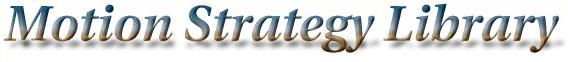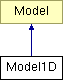Main Page   Namespace List   Class Hierarchy   Compound List   File List   Compound Members   File Members

# Model1D Class Reference

A simple one-dimensional model for dynamics studies. More...

`#include <modelmisc.h>`

Inheritance diagram for Model1D:List of all members.

## Public Methods

Model1D (string path)
virtual ~Model1D ()
virtual MSLVector StateToConfiguration (const MSLVector &x)
A method that converts a Model state in to a Geom configuration. More...

virtual MSLVector Integrate (const MSLVector &x, const MSLVector &u, const double &h)
Perform integration from state x, using input u, over time step h. More...

virtual MSLVector StateTransitionEquation (const MSLVector &x, const MSLVector &u)
The state transition equation, or equations of motion, xdot=f(x,u). More...

virtual double Metric (const MSLVector &x1, const MSLVector &x2)
A distance metric, which is Euclidean in the base class. More...

double Force

## Detailed Description

A simple one-dimensional model for dynamics studies.

## Constructor & Destructor Documentation

 Model1D::Model1D ( string path )

 virtual Model1D::~Model1D ( ) ` [inline, virtual]`

## Member Function Documentation

 MSLVector Model1D::Integrate ( const MSLVector & x, const MSLVector & u, const double & h ) ` [virtual]`
 Perform integration from state x, using input u, over time step h. Implements Model.

 double Model1D::Metric ( const MSLVector & x1, const MSLVector & x2 ) ` [virtual]`
 A distance metric, which is Euclidean in the base class. Reimplemented from Model.

 MSLVector Model1D::StateToConfiguration ( const MSLVector & x ) ` [virtual]`
 A method that converts a Model state in to a Geom configuration. Reimplemented from Model.

 MSLVector Model1D::StateTransitionEquation ( const MSLVector & x, const MSLVector & u ) ` [virtual]`
 The state transition equation, or equations of motion, xdot=f(x,u). Implements Model.

## Member Data Documentation

 double Model1D::Force

The documentation for this class was generated from the following files: Motion Strategy Library

Web page maintained by Steve LaValle